Trending Today#### Fidelity Bank 2019Q1: Better Earnings, Stronger Valuation, ROI ProspectsCoy: Fidelity Bank Plc
Rating: Hold
Current Market Price: N1.72
NPL RATIO: 4.9%
Year High: N2.90
Year Low: N1.61
Fair Value: N2.67
Equity Analyst: Jeariogbe Tunde Segun

Introduction
• This report observed the financial performance statistics of Fidelity Bank Plc for the first three months of 2019, compared the same with the similar quarter of 2018 and establish growth and financial status.
• We also observed few valuation metrics from its full-year performance indices for the year ended 31st December 2019.
• Generally, the result is impressive when measured side by side with figures released in the similar quarter of 2018.
• Although there were few differences in restated figures, we rated the earnings quality Good.
• Non-performing loan for the period improved from the 5.7% posted in 2018 first quarter to 4.9% at the end of the first three months of 2019.
• See below for other financial indices comparison.• Gross Earnings for the quarter was estimated at N48.44 billion, this is 11.81% above the N43.32 billion posted in the corresponding period of 2018.
• Interest Income stood at N38.67 billion, as against the N37.68 billion of 2018.
• Interest Expenses, on the other hand, improved by 6.52% to N22.90 billion, compared to N21.49 billion of the 2018 Q1.
• Net Interest Income, therefore, dropped by 4.78% to N14.73 billion, from N15.49 billion.
• Operating Expenses stood at N15.69 billion, 9.39% above the previous N14.35 billion.
• Profit before Tax is currently estimated at N6.67 billion, a growth of 33.96% above the N4.98 billion achieved in 2018 first quarter.
• Having considered the N734 million Tax Expenses, estimated profit for the year stood at N5.93 billion, representing 28.36% increase over the N4.62 billion posted at the end of 2018Q1.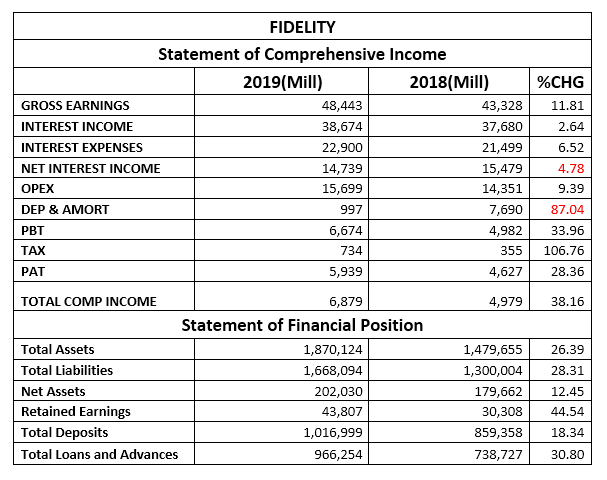• Total Assets improved by26.39% to N1.87 trillion, compared to the N1.47 trillion at the end of 2018 first quarter.
• In a similar trend, Total Liabilities grew by 28.31% to N1.66 trillion, up from the N1.30 trillion reported in 2018.
• Net Assets improved to N202.03 billion, against N179.66 billion in 2018. The difference between both figures is the same as a 12.45% drop.
• Retained Earnings at the end of the period is estimated at N43.80 billion, which is 44.54% appreciation over the N30.30 billion stated in its 2018 first quarter financials.
• Total Deposits achieved through the first three months of 2019 grew by 18.34% to N1.01 trillion, compared to N859.35 billion in 2018 first quarter.
• Total Loans and Advances stood over a comparable period by 30.80% at the current value of N966.25 billion, up from N738.72 billion in 2018.

Financial Strength/Solvency Ratios
• Debt Ratio which compared both Total Assets to Total Liabilities stood at 0.89x or 89%, implying that the Total Liabilities is 89% of Total Assets. Please understand that Total Liabilities is inclusive of Total Deposits for the period.
• Total Debt to Equity for the quarter stood at 8.26x, same as 14.11% above the 7.24x estimated in 2018. This implies that the company used debt equivalent to 8.26 times of its equity through the period (note debt used is total liabilities and includes total deposits for the period).
• Equity Ratio which compared Equity to Total Assets stood at 0.11x as against 0.12x, implying that the reported Equity at the end of the period is 11% of Total Assets.
• Estimated Beta value for Fidelity Bank as at the time this report was compiled stood at 1.34, which is above market beta though below the 1.50 industry average.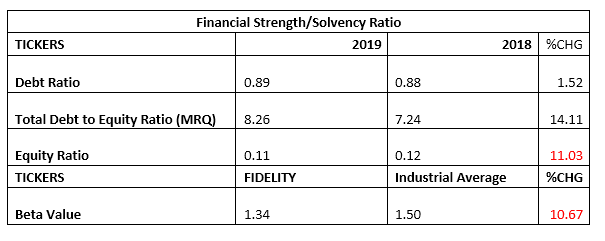Profitability Ratios
• EBITDA margin estimated for the period stood at 34.00%, 37.38% below the 54.29% estimated from the corresponding quarters’ numbers.
• Pre-Tax Margin improved by 19.82% to 13.78% against the 11.50% estimated at the end of 2018 first quarter.
• Interest Expenses to Gross Earnings dipped by 4.73% to 47.27%, compared to the previously estimated 49.62%.
• Return on Average Equity through the first three months of 2019 was 2.94%, up from 2.58% estimated from 2018 numbers.
• See table below for detailed profitability ratios and comparison.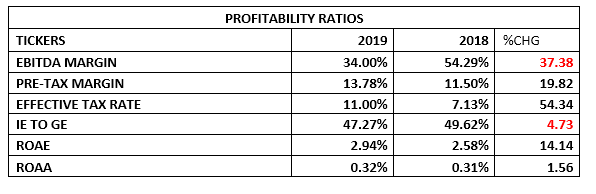Efficiency Ratios
• Operating Expenses in the period is estimated to be 32.41% of the estimated Gross Earnings, which is below the 33.12% achieved in 2018 first quarter financials. Please note that this implies marginal adjustments in the rate of expenses.
• Gross Earnings estimated from the bank’s financials is same as 2.59% (2018Q1-2.93%) of the Total Assets value posted for the period under analysis.
• Total Loan and Advances posted for the quarter is 95.01% of Total Deposit, this is 10.53% improvement over the 85.96% estimate in 2018 first quarter.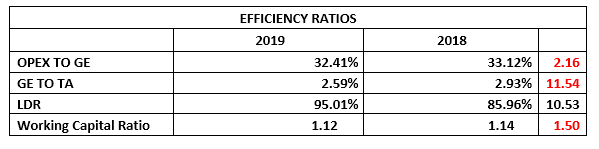Investment/Valuation Ratios
• The profit earned per unit of Fidelity Bank is estimated at N0.20, representing 28.36% improvement over the N0.16 reported in the similar quarter of 2018.
• Total Comprehensive Income is estimated at N0.24, which is 38.16% above the N0.17 TCI/share achieved in 2018.
• P/E Ratio dropped to 2.21x from 3.99x, which represents a drop in the period to recoup the investment in its shares.
• The said earnings per share is a yield of 11.32% of the price of each share of Fidelity Bank on the floor of the Nigerian Stock Exchange on the day the result was unveiled to the investing public.
• The estimated value of each unit of Fidelity Bank in its book is N6.97, which is a 12.45% improvement over that of previously estimated N6.20.
• Confirming the academic under-priced position of Fidelity Bank’s shares on the exchange is the Price to Book Value estimate that stands far below unity.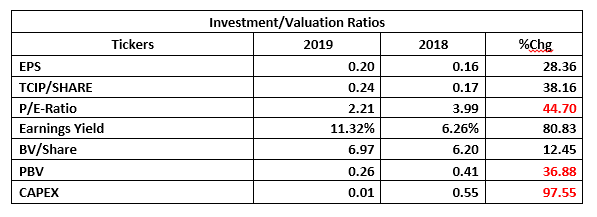Valuation
Exploring our blend of valuation tools, we conservatively valued each units of Fidelity Bank shares at N2.67, thus, we rated it as Hold.

## Market Update